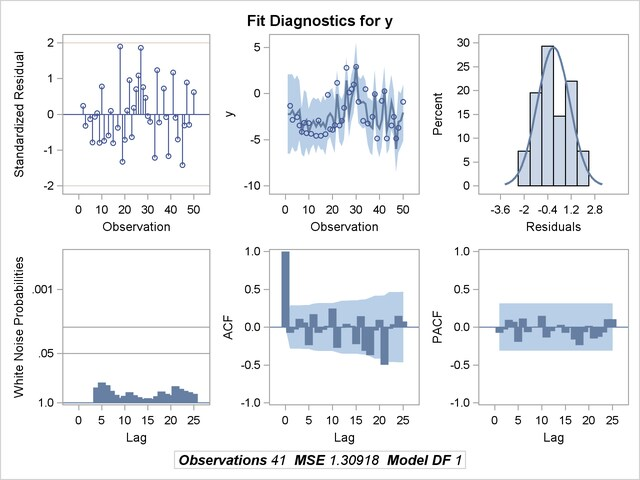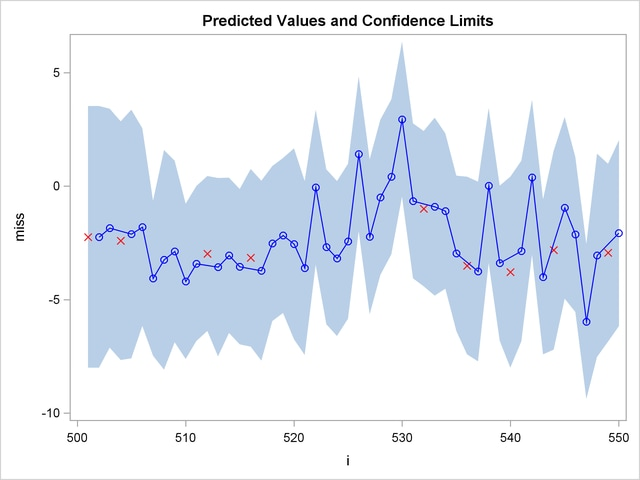The AUTOREG Procedure

## Example 8.4 Missing Values

In this example, a pure autoregressive error model with no regressors is used to generate 50 values of a time series. Approximately 15% of the values are randomly chosen and set to missing. The following statements generate the data:

```title  'Simulated Time Series with Roots:';
title2 ' (X-1.25)(X**4-1.25)';
title3 'With 15% Missing Values';
data ar;
do i=1 to 550;
e = rannor(12345);
n = sum( e, .8*n1, .8*n4, -.64*n5 );  /* ar process  */
y = n;
if ranuni(12345) > .85 then y = .;    /* 15% missing */
n5=n4; n4=n3; n3=n2; n2=n1; n1=n;     /* set lags    */
if i>500 then output;
end;
run;
```

The model is estimated using maximum likelihood, and the residuals are plotted with 99% confidence limits. The PARTIAL option prints the partial autocorrelations. The following statements fit the model:

```proc autoreg data=ar partial;
model y = / nlag=(1 4 5) method=ml;
output out=a predicted=p residual=r ucl=u lcl=l alphacli=.01;
run;
```

The printed output produced by the AUTOREG procedure is shown in Output 8.4.1 and Output 8.4.2. Note: the plot Output 8.4.2 can be viewed in the Autoreg.Model.FitDiagnosticPlots category by selecting ViewResults.

Output 8.4.1 Autocorrelation-Corrected Regression Results
 Simulated Time Series with Roots: (X-1.25)(X**4-1.25) With 15% Missing Values

The AUTOREG Procedure

Dependent Variable y

Ordinary Least Squares Estimates
SSE 182.972379 DFE 40
MSE 4.57431 Root MSE 2.13876
SBC 181.39282 AIC 179.679248
MAE 1.80469152 AICC 179.781813
MAPE 270.104379 HQC 180.303237
Durbin-Watson 1.3962 Regress R-Square 0.0000
Total R-Square 0.0000

Parameter Estimates
Variable DF Estimate Standard Error t Value Approx
Pr > |t|
Intercept 1 -2.2387 0.3340 -6.70 <.0001

Estimates of Autocorrelations
Lag Covariance Correlation -1 9 8 7 6 5 4 3 2 1 0 1 2 3 4 5 6 7 8 9 1
0 4.4627 1.000000 |                    |********************|
1 1.4241 0.319109 |                    |******              |
2 1.6505 0.369829 |                    |*******             |
3 0.6808 0.152551 |                    |***                 |
4 2.9167 0.653556 |                    |*************       |
5 -0.3816 -0.085519 |                  **|                    |

Partial Autocorrelations
1 0.319109
4 0.619288
5 -0.821179

 Preliminary MSE 0.7609

Estimates of Autoregressive Parameters
Lag Coefficient Standard Error t Value
1 -0.733182 0.089966 -8.15
4 -0.803754 0.071849 -11.19
5 0.821179 0.093818 8.75

Expected Autocorrelations
Lag Autocorr
0 1.0000
1 0.4204
2 0.2480
3 0.3160
4 0.6903
5 0.0228

 Algorithm converged.

Maximum Likelihood Estimates
SSE 48.4396756 DFE 37
MSE 1.30918 Root MSE 1.14419
SBC 146.879013 AIC 140.024725
MAE 0.88786192 AICC 141.135836
MAPE 141.377721 HQC 142.520679
Durbin-Watson 2.9457 Regress R-Square 0.0000
Total R-Square 0.7353

Parameter Estimates
Variable DF Estimate Standard Error t Value Approx
Pr > |t|
Intercept 1 -2.2370 0.5239 -4.27 0.0001
AR1 1 -0.6201 0.1129 -5.49 <.0001
AR4 1 -0.7237 0.0914 -7.92 <.0001
AR5 1 0.6550 0.1202 5.45 <.0001

Expected Autocorrelations
Lag Autocorr
0 1.0000
1 0.4204
2 0.2423
3 0.2958
4 0.6318
5 0.0411

Autoregressive parameters assumed given
Variable DF Estimate Standard Error t Value Approx
Pr > |t|
Intercept 1 -2.2370 0.5225 -4.28 0.0001

Output 8.4.2 Diagnostic PlotsThe following statements plot the residuals and confidence limits:

```data reshape1;
set a;
miss = .;
if r=. then do;
miss = p;
p = .;
end;
run;

title 'Predicted Values and Confidence Limits';

proc sgplot data=reshape1 NOAUTOLEGEND;
band x=i upper=u lower=l;
scatter y=miss x=i/ MARKERATTRS =(symbol=x color=red);
series y=p x=i/markers MARKERATTRS =(color=blue) lineattrs=(color=blue);
run;
```

The plot of the predicted values and the upper and lower confidence limits is shown in Output 8.4.3. Note that the confidence interval is wider at the beginning of the series (when there are no past noise values to use in the forecast equation) and after missing values where, again, there is an incomplete set of past residuals.

Output 8.4.3 Plot of Predicted Values and Confidence IntervalPrevious Page | Next Page | Top of Page